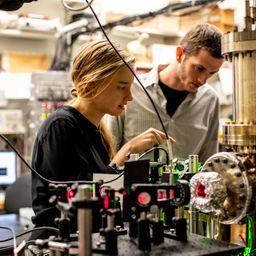## a. 10^{-7} : \text { ultraviolet }b. 10^{-10} \mathrm{m} : \text { xray }

Quantum Physics

Atomic Physics

### Discussion

You must be signed in to discuss.
##### Top Physics 103 Educators
LB##### Marshall S.

University of Washington##### Aspen F.

University of Sheffield

### Video Transcript

Okay, so in this problem, we're talking about lightning, and we're told it produces a max air temperature. 10 of the four Calvin while it's Reed. Hi. Um, and the nuclear's explosions, or 10 of the seven color been. So we want to figure out basically what color the radiation is from these two processes. And we want to use wise Displacement Law, which says that the Lambda Max of the emitted radiation times the temperature and units of Calvin looks is equal to 0.298 C was the number. Excuse me to 898 The double check on that. I don't have that constant memorized, but I guess it's not so bad. 8980.2 eight 98 times 10 to the minus two kelvin meter. And then you want assault for Lambda so you could just divide both sides by the temperature. So I'll remove this, bring it to the other side to fighting them, but dividing it out. And so I'm gonna calculate that in the case of one, temperature equals tend to the four Calvin. So let's see what I get. I bet that this is gonna be I think this is gonna be visible, I guess, because it's lightning. So we would expect to see to see something in the visible spectrum. But let's go ahead and find out what we get. So plucking this into calculator on a separate screen, so it's kind of a four caldron. So that's 200. Oh, I guess I could have just done that in my head. That's funny. Um, 289.8. Mmm. We'll do the next one in my head. T So that plane's Lambda equals, um, 200 89.8, um, nanometers. So I would say that that you be so that's purple. Oh, that's my lightning's purple. That's so interesting. Um, and then for a nuclear explosion who? I guess that's, um I never thought I would that way. That's why it's, um it's a ionizing radiation. So if you do plug in 10 of the seven here, then it's gonna be 70.2 89 a, uh, times 10 to the minus nine. So then that's nano meters. I think that's in the X ray spectrum. So, um, look at yeah, 0.1 toe, 10 nano meters. So I'd say that that's an X rayUniversity of Washington

#### Topics

Quantum Physics

Atomic Physics

##### Top Physics 103 Educators
LB##### Marshall S.

University of Washington##### Aspen F.

University of Sheffield